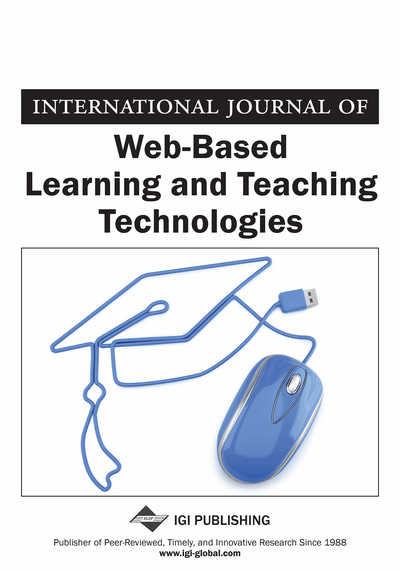# Automatic Semantic Generation and Arabic Translation of Mathematical Expressions on the Web

DOI: 10.4018/jwltt.2013010101
OnDemand:
(Individual Articles)
Available
\$29.50
No Current Special Offers

## Abstract

Automatic processing of mathematical information on the web imposes some difficulties. This paper presents a novel technique for automatic generation of mathematical equations semantic and Arabic translation on the web. The proposed system facilitates unambiguous representation of mathematical equations by correlating equations to their known names. The ability to extract the equation meaning from its structure is vital when searching for mathematical equations. The general structure of the equation is recognized to identify the equation meaning. On the other hand, people who cannot understand Latin script notation of mathematical expressions have difficulty when they try to read them on the web as it is available mostly in Latin. Arabic mathematical expressions flow from right to left and they use specific symbols. The proposed system automatically translates the mathematical equations from Latin to Arabic. This translation can be combined with the text translation of mathematical web contents (generated by online tools) to be recognized by the people who understand only Arabic text. The proposed system is implemented using Java and it is evaluated using a set of web pages with MathML contents which is rendered in Mozilla web browser.
Article Preview
Top

## 1. Introduction

Mathematical notations are used in mathematics, physics, engineering, and economics. These notations include symbolic representation, such as numbers, function symbols (e.g., sin, tan….), operators (e.g., +, *…..), conceptual symbols and variables (e.g., lim, dy/dx). These notations form a mathematical expression.

MathML is a standard XML-based encoding for mathematical structures (Carlisle et al., 2003b). The MathML elements can be embedded inside XHTML documents. All popular web browsers can display MathML equations. As XML supports Unicode, there are no difficulties with using Arabic letters in MathML. MathML is intended to provide a clear presentation of mathematics instead of using images of the expressions as these images are difficult to see, read, and search (Eddahibi et al., 2004). MathML allow the mathematical contents to be rendered, received, and processed on the web in a way similar to HTML.

A large number of learners are concerned about the availability Arabic mathematical contents online (Banouni et al., 2004; Lazrek et al., 2006). Large number of websites resources use Latin notation to present mathematical contents. The users who cannot understand English use online translation services to convert the contents in these learning resources into Arabic language. Unfortunately, the mathematical equations are not translated into the Arabic equivalent mathematical notation.

Processing mathematical expressions on the web is not an easy work (Su et al., 2008), since these expressions have special requirements like providing correct visual appearance of expressions on the web and encoding their semantic. Encoding the semantic of mathematical expressions means the encoding of the general structure of the expression, which is used in many applications to obtain the actual expression or to search for math contents (Carlisle et al., 2003).

Wang et al. (2003) presented a work about semantic of mathematics on the web called Web for Mathematical Education (WME). Another work is EditMath presented by Su et al. (2008) which provide online framework to present mathematics, and support the use of semantic features to encode and process mathematical expressions.

Searching for mathematical equations has many problems as the structure of equations may have different representations such as the coefficient notations (e.g.,and nCk). Understanding the meaning of mathematical expressions will support the searching of these expressions. For example, the equationdescribes the distance between two points.

As described in Kohlhase and Sucan (2006), there are many difficulties when searching of mathematical expressions: first, the same mathematical symbols can be used in different forms to denote the same expression (e.g.,and nCk denotes the same concept but in different forms). Second, the same mathematical expression has many different interpretations (e.g.,can refer to a Rieman Integral or a Lebesgue Integral). Third, an expression contains a set of variables maybe replicated by just renaming the variables (e.g.,has the same meaning as).

The Arabic language is the official language for the countries in the Middle East and North Africa. The Arabic script is used in extended version by other major languages. For example, Urdu (Pakistan), Persian and Farsi (Iran, India), Berber (North Africa), Sindhi (India), Uyghur, Kirgiz (Central Asia), Pashtun (Afghanistan), Kurdish, Jawi, Baluchi, and several African languages.

## Complete Article List

Search this Journal:
Reset
Volume 18: 2 Issues (2023)
Volume 17: 8 Issues (2022)
Volume 16: 6 Issues (2021)
Volume 15: 4 Issues (2020)
Volume 14: 4 Issues (2019)
Volume 13: 4 Issues (2018)
Volume 12: 4 Issues (2017)
Volume 11: 4 Issues (2016)
Volume 10: 4 Issues (2015)
Volume 9: 4 Issues (2014)
Volume 8: 4 Issues (2013)
Volume 7: 4 Issues (2012)
Volume 6: 4 Issues (2011)
Volume 5: 4 Issues (2010)
Volume 4: 4 Issues (2009)
Volume 3: 4 Issues (2008)
Volume 2: 4 Issues (2007)
Volume 1: 4 Issues (2006)
View Complete Journal Contents Listing